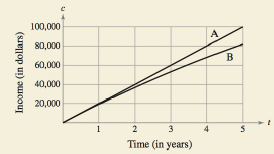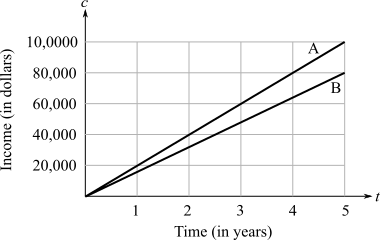Chapter 6.1, Problem 78E### Calculus: An Applied Approach (Min...

10th Edition
Ron Larson
ISBN: 9781305860919

#### Solutions

Chapter
Section### Calculus: An Applied Approach (Min...

10th Edition
Ron Larson
ISBN: 9781305860919
Textbook Problem
1 views

# HOW DO YOU SEE IT? The graphs of two equations show the expected income and the present value of the expected income for a company. Which graph represents the expected income and which graph represents the present value of the expected income? Explain your reasoning.To determine

The graphs which represents the expected income and present value respectively.

The provided graphs of two equations is shown below:Explanation

Given Information:

The provided graph is shown below:

Consider the provided graph where x represents the time in years and y represents the income in dollars.

First understand the present value:

Present value is the amount which have to deposited today to produce the future payment. Present value can not be more than the future value...

### Still sussing out bartleby?

Check out a sample textbook solution.

See a sample solution

#### The Solution to Your Study Problems

Bartleby provides explanations to thousands of textbook problems written by our experts, many with advanced degrees!

Get Started

#### Find more solutions based on key concepts